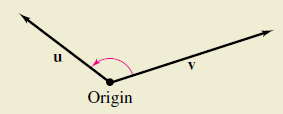Chapter 11.3, Problem 50E

Chapter
Section
Textbook Problem

# HOW DO YOU SEE IT? What is known about θ , the angle between two nonzero vectors u and v, when(a) u ⋅ v = 0 ? (b) u ⋅ v > 0 ? (c) u ⋅ v < 0 ?(a)

To determine

To Calculate: θ the angle between two non-zero vectors u and v.

Explanation

Given:

u.v=0

Formula Used:

θ=arccos(u.vuv)</

(b)

To determine

To Calculate: θ the angle between two non-zero vectors u and v, when u.v > 0

(c)

To determine

To Calculate: θ the angle between two non-zero vectors u and v, when uv<0

### Still sussing out bartleby?

Check out a sample textbook solution.

See a sample solution

#### The Solution to Your Study Problems

Bartleby provides explanations to thousands of textbook problems written by our experts, many with advanced degrees!

Get Started

#### Find the median for the following set of scores: 1, 9, 3, 6, 4, 3, 11, 10

Essentials of Statistics for The Behavioral Sciences (MindTap Course List)

#### In Exercises 33-38, rewrite the expression using positive exponents only. 37. 120(s + t)3

Applied Calculus for the Managerial, Life, and Social Sciences: A Brief Approach

#### Sometimes, Always, or Never: If f(x) is continuous on [a, b], then abf(x) dx exists.

Study Guide for Stewart's Single Variable Calculus: Early Transcendentals, 8th